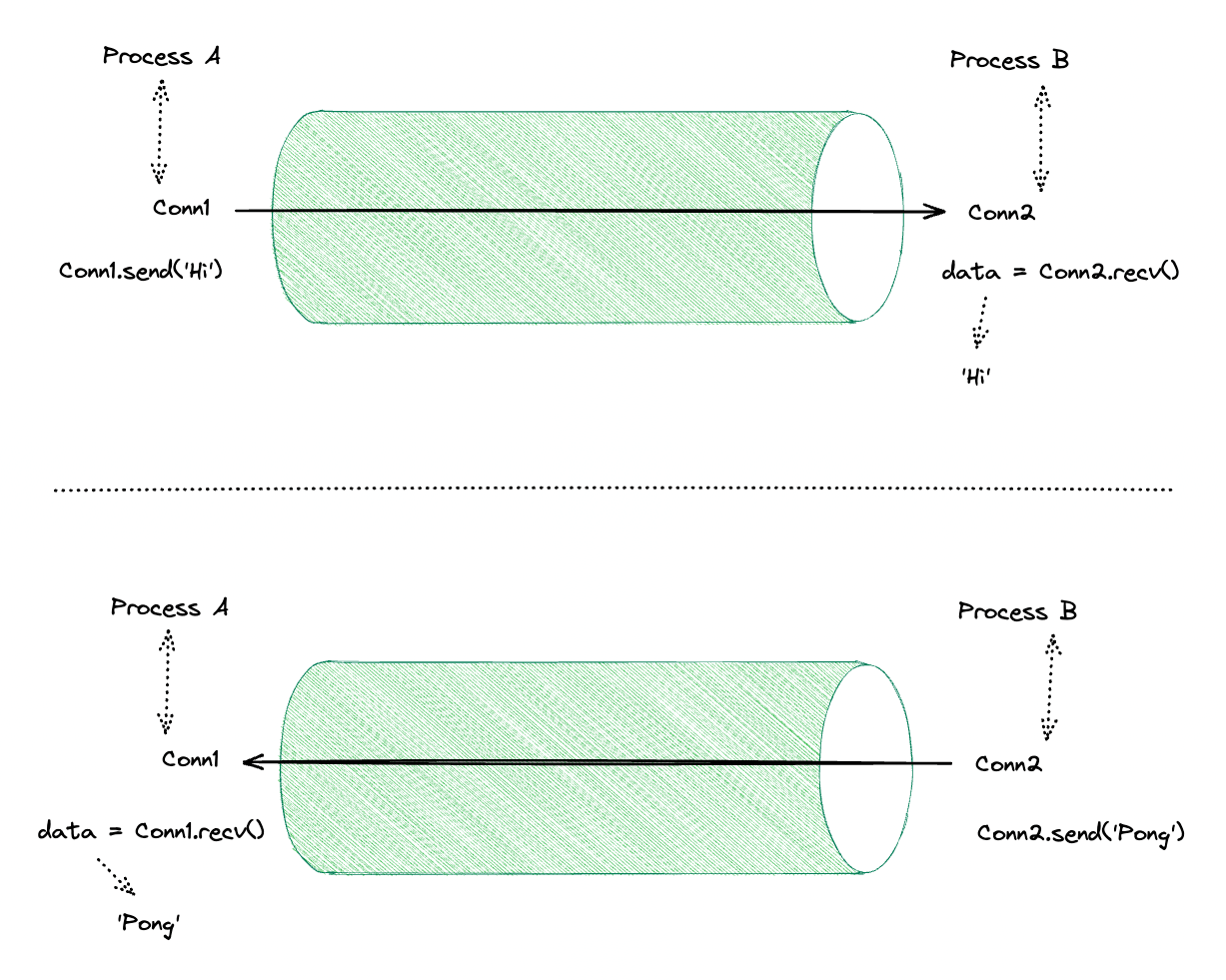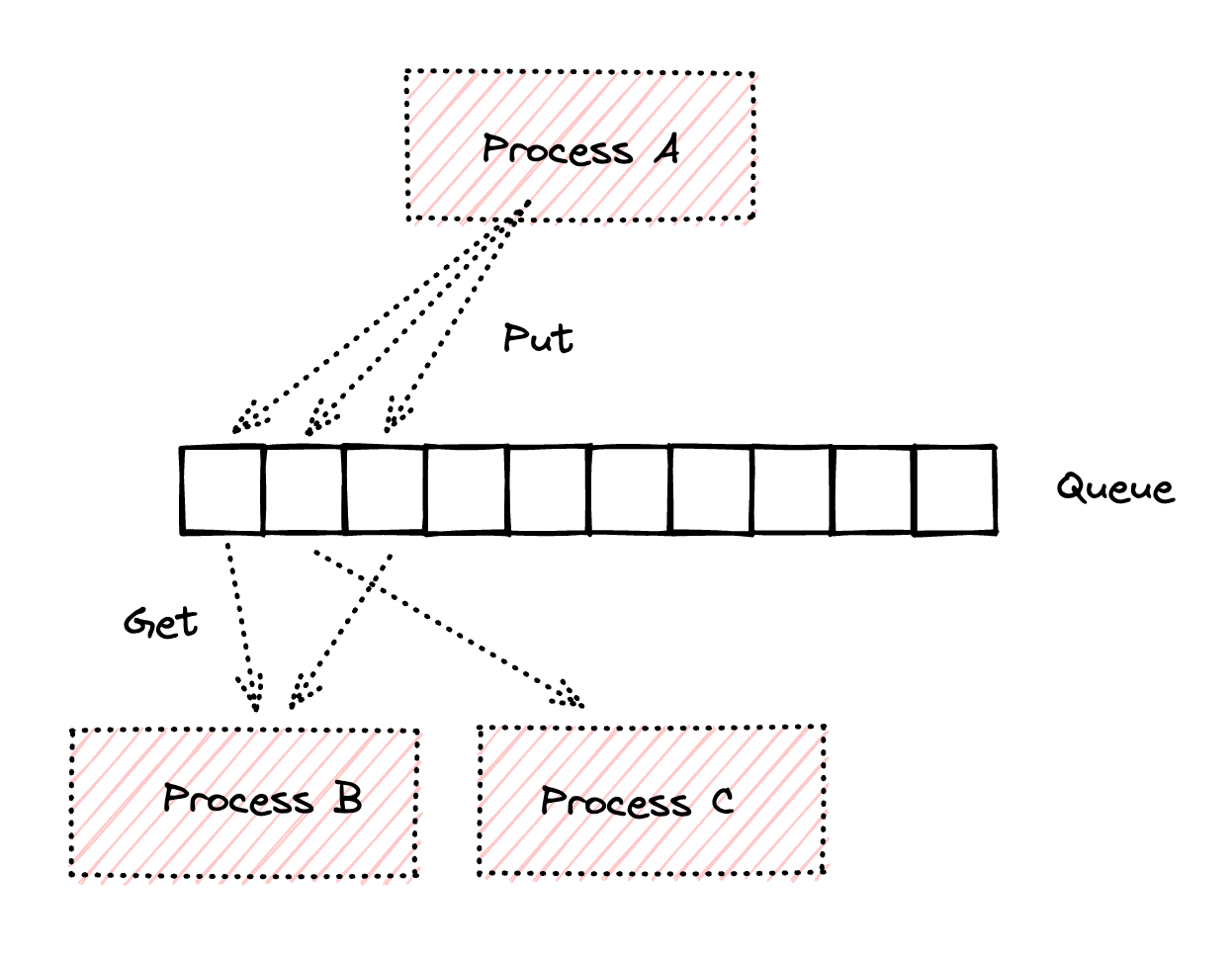# multiprocessing 模組進階篇 - Pipe, Queue, Array, RawArray 以及 Structure 之教學範例

Posted on  Aug 7, 2023  in  Python 程式設計 - 中階  by  Amo Chen  ‐ 5 min read

• 以參數(args)的方式傳遞資料
• 以共享記憶體(Shared Memory)中的 Value 物件傳遞資料
• 以 fork 方式傳遞資料， fork 出來的子 process 會繼承父 process 的資源，所以可以存取原本父 process 內的資料
• 透過 Manager 共享資料， Manager 會在一個稱為 server process 的 process 中管理共享的資料，並且代理其他 process 操作這些共享資料

• Python 3

### Pipe #``````import os

from multiprocessing import Process, Pipe

def do_job(conn):
pid = os.getpid()
while True:
i = conn.recv()
if i is None:
print(f'{pid}| Nothing to do. Exit.', flush=True)
conn.send(None)
conn.close()
break
print(f'{pid}| Got {i} from the pipe.', flush=True)
conn.send(i + 5)

if __name__ == '__main__':
pid = os.getpid()
conn1, conn2 = Pipe()

p = Process(target=do_job, args=(conn2, ))
p.start()

for i in range(1, 11):
conn1.send(i)
conn1.send(None)

while True:
result = conn1.recv()
if result is None:
conn1.close()
print(f'{pid}| Close the pipe', flush=True)
break
print(f'{pid}| Receive {result} from the pipe', flush=True)
``````

``````7985| Got 1 from the pipe.
7985| Got 2 from the pipe.
7985| Got 3 from the pipe.
7985| Got 4 from the pipe.
7985| Got 5 from the pipe.
7985| Got 6 from the pipe.
7985| Got 7 from the pipe.
7985| Got 8 from the pipe.
7985| Got 9 from the pipe.
7934| Receive 6 from the pipe
7985| Got 10 from the pipe.
7934| Receive 7 from the pipe
7985| Nothing to do. Exit.
7934| Receive 8 from the pipe
7934| Receive 9 from the pipe
7934| Receive 10 from the pipe
7934| Receive 11 from the pipe
7934| Receive 12 from the pipe
7934| Receive 13 from the pipe
7934| Receive 14 from the pipe
7934| Receive 15 from the pipe
7934| Close the pipe
``````

### Queue #

Queue 是用 Pipe 與 locs/semaphores 實作而成，與 Pipe 不同的是：

• Queue 可以設定 queue size, 預設為無限制
• Queue 可以同時給多個 Processes 存取``````import os

from multiprocessing import Process, Queue

def do_job(queue):
pid = os.getpid()
while True:
data = queue.get()
if data is None:
print(f'{pid}| Nothing to do. Exit.', flush=True)
break
print(f'{pid}| Got {data} from the queue.', flush=True)

if __name__ == '__main__':
queue = Queue()

processes = [
Process(target=do_job, args=(queue, ))
for _ in range(2)
]

for p in processes:
p.start()  # 執行 process

for i in range(1, 21):
queue.put(i)  # 放入資料

queue.put(None)
queue.put(None)

for p in processes:
p.join()  # 等待 process 執行結束
``````

p.s. queue.get() 也可以設定 timeout (單位為秒，必須為正整數）。

``````4394| Got 1 from the queue.
4395| Got 2 from the queue
4394| Got 3 from the queue
4395| Got 4 from the queue
4394| Got 5 from the queue
4395| Got 6 from the queue
4394| Got 7 from the queue
4395| Got 8 from the queue
4394| Got 9 from the queue
4395| Got 10 from the queue
4394| Got 11 from the queue
4395| Got 12 from the queue
4394| Got 13 from the queue
4395| Got 14 from the queue
4394| Got 15 from the queue
4395| Got 16 from the queue
4394| Got 17 from the queue
4395| Got 18 from the queue
4394| Got 19 from the queue
4395| Got 20 from the queue
4394| Nothing to do. Exit.
4395| Nothing to do. Exit.
``````

### Array #

``````import os
import random

from multiprocessing import Process, Array

def do_job(arr):
pid = os.getpid()
print(f'{pid}| Got the Array')
for i in range(len(arr)):
arr.acquire()
arr[i] = random.randint(0, 100)
arr.release()

if __name__ == '__main__':
pid = os.getpid()
arr = Array('d', *10)

print(f'{pid}| Pass the Array')
p = Process(target=do_job, args=(arr, ))
p.start()
p.join()

print(f'{pid}| Print the Array')
for i in arr:
print(f'{pid}| {i}')
``````

``````10108| Pass the Array
10159| Got the Array
10108| Print the Array
10108| 71.0
10108| 66.0
10108| 78.0
10108| 24.0
10108| 87.0
10108| 0.0
10108| 54.0
10108| 0.0
10108| 50.0
10108| 45.0
``````

### RawArray #

Note that setting and getting an element is potentially non-atomic – use Array() instead to make sure that access is automatically synchronized using a lock.

RawArray 與 Array 相似，用於 IPC 以傳遞多個相同型態(type)的資料，但是 RawArray 並不保證寫入、讀取具備原子性(atomic), 也沒有 `acquire()` , `release()` 等 Lock 相關方法可以呼叫，如果多個 Process 同時存取，很高機率會有 race condition 問題發生。

``````import os
import random

from multiprocessing import Process, RawArray

def do_job(array):
pid = os.getpid()
print(f'{pid}| Got the RawArray')
for i in array:
print(f'{pid}| {i}')

if __name__ == '__main__':
pid = os.getpid()
arr = RawArray('d', [random.randint(0, 100) for _ in range(10)])

print(f'{pid}| Pass the RawArray')
p = Process(target=do_job, args=(arr, ))
p.start()
p.join()
``````

``````9317| Pass the RawArray
9368| Got the RawArray
9368| 41.0
9368| 51.0
9368| 73.0
9368| 85.0
9368| 8.0
9368| 92.0
9368| 91.0
9368| 6.0
9368| 43.0
9368| 14.0
``````

p.s. Value 也有 1 個相似的 RawValue 可以使用， RawValue 與 RawArray 同樣不保證原子性(atomic)

### 定義複雜的資料型態 - Structure #

``````from ctypes import Structure, c_ushort, c_wchar

class User(Structure):
_fields_ = [
('name', c_wchar * 5),
('age', c_ushort),
]
``````

``````import os

from multiprocessing import Process, RawArray
from ctypes import Structure, c_ushort, c_wchar

class User(Structure):
_fields_ = [
('name', c_wchar * 5),
('age', c_ushort),
]

def do_job(arr):
pid = os.getpid()
print(f'{pid}| Got the array', flush=True)
for i in arr:
print(f'{pid}| Name: {i.name}, Age: {i.age}', flush=True)
print(f'{pid}| Exit', flush=True)

if __name__ == '__main__':
arr = RawArray(User, [('Joe', 18, ), ('Nemo', 9, )])
p = Process(target=do_job, args=(arr, ))
p.start()
p.join()
``````

``````27208| Got the array
27208| Name: Joe, Age: 18
27208| Name: Nemo, Age: 9
27208| Exit
``````

### 總結 #

multiprocessing — Process-based parallelism

ctypes — A foreign function library for Python

7 Ways to Share a Numpy Array Between Processes